Adaptation measures are defined by parameters that alter the exposures, hazard or impact functions. Risk transfer options are also considered. Single measures are defined in the Measure class, which can be aggregated to a MeasureSet.

## Measure class#

A measure is characterized by the following attributes:

Related to measure’s description:

• name (str): name of the action

• haz_type (str): related hazard type (peril), e.g. TC

• color_rgb (np.array): integer array of size 3. Gives color code of this measure in RGB

• cost (float): discounted cost (in same units as assets). Needs to be provided by the user. See the example provided in climada_python/climada/data/system/entity_template.xlsx sheets _measures_details and _discounting_sheet to see how the discounting is done.

Related to a measure’s impact:

• hazard_set (str): file name of hazard to use

• hazard_freq_cutoff (float): hazard frequency cutoff

• exposure_set (str): file name of exposure to use

• hazard_inten_imp (tuple): parameter a and b of hazard intensity change

• mdd_impact (tuple): parameter a and b of the impact over the mean damage degree

• paa_impact (tuple): parameter a and b of the impact over the percentage of affected assets

• imp_fun_map (str): change of impact function id, e.g. ‘1to3’

• exp_region_id (int): region id of the selected exposures to consider ALL the previous parameters

• risk_transf_attach (float): risk transfer attachment. Applies to the whole exposure.

• risk_transf_cover (float): risk transfer cover. Applies to the whole exposure.

Paramters description:

hazard_set and exposures_set provide the file names in h5 format (generated by CLIMADA) of the hazard and exposures to use as a result of the implementation of the measure. These might be further modified when applying the other parameters.

hazard_inten_imp, mdd_impact and paa_impact transform the impact functions linearly as follows:

 intensity = intensity*hazard_inten_imp + hazard_inten_imp
mdd = mdd*mdd_impact + mdd_impact
paa = paa*paa_impact + paa_impact


hazard_freq_cutoff modifies the hazard by putting 0 intensities to the events whose impact exceedance frequency are greater than hazard_freq_cutoff.

imp_fun_map indicates the ids of the impact function to replace and its replacement. The impf_XX variable of Exposures with the affected impact function id will be correspondingly modified (XX refers to the haz_type of the measure).

exp_region_id will apply all the previous changes only to the region_id indicated. This means that only the exposures with that region_id and the hazard’s centroids close to them will be modified with the previous changes, the other regions will remain unaffected to the measure.

risk_transf_attach and risk_transf_cover are the deductible and coverage of any event to happen.

Methods description:

The method check() validates the attibutes. apply() applies the measure to a given exposure, impact function and hazard, returning their modified values. The parameters related to insurability (risk_transf_attach and risk_transf_cover) affect the resulting impact and are therefore not applied in the apply() method yet.

calc_impact() calls to apply(), applies the insurance parameters and returns the final impact and risk transfer of the measure. This method is called from the CostBenefit class.

The method apply() allows to visualize the effect of a measure. Here are some examples:

# effect of mdd_impact, paa_impact, hazard_inten_imp
%matplotlib inline
import numpy as np
from climada.entity import ImpactFuncSet, ImpfTropCyclone, Exposures

# define measure
meas = Measure(
name='Mangrove',
haz_type='TC',
color_rgb=np.array([1, 1, 1]),
cost=500000000,
mdd_impact=(1, 0),
paa_impact=(1, -0.15),
hazard_inten_imp=(1, -10), # reduces intensity by 10
)

# impact functions
impf_tc = ImpfTropCyclone.from_emanuel_usa()
impf_all = ImpactFuncSet([impf_tc])
impf_all.plot();

# dummy Hazard and Exposures
haz = Hazard('TC') # this measure does not change hazard
exp = Exposures() # this measure does not change exposures

# new impact functions
new_exp, new_impfs, new_haz = meas.apply(exp, impf_all, haz)
axes = new_impfs.plot();
axes.set_title('TC: Modified impact function')

Text(0.5, 1.0, 'TC: Modified impact function')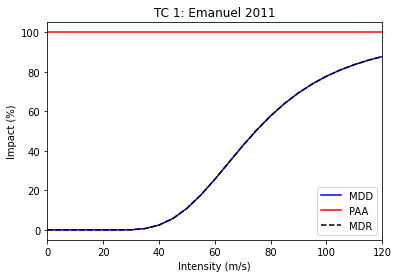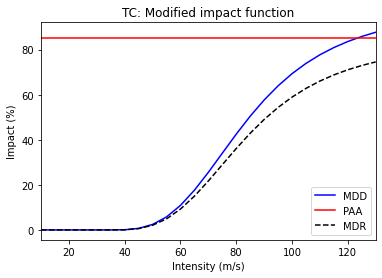# effect of hazard_freq_cutoff
import numpy as np
from climada.entity import ImpactFuncSet, ImpfTropCyclone, Exposures

# define measure
meas = Measure(
name='Mangrove',
haz_type='TC',
color_rgb=np.array([1, 1, 1]),
cost=500000000,
hazard_freq_cutoff=0.0255,
)

# impact functions
impf_tc = ImpfTropCyclone.from_emanuel_usa()
impf_all = ImpactFuncSet([impf_tc])

# Hazard
haz = Hazard.from_hdf5(HAZ_DEMO_H5)
haz.check()

# Exposures
exp = Exposures.from_hdf5(EXP_DEMO_H5)
exp.check()

# new hazard
new_exp, new_impfs, new_haz = meas.apply(exp, impf_all, haz)
# if you look at the maximum intensity per centroid: new_haz does not contain the event with smaller impact (the most frequent)
haz.plot_intensity(0);
new_haz.plot_intensity(0);
# you might also compute the exceedance frequency curve of both hazard
imp = ImpactCalc(exp, impf_all, haz).impact()
ax = imp.calc_freq_curve().plot(label='original');

new_imp = ImpactCalc(new_exp, new_impfs, new_haz).impact()
new_imp.calc_freq_curve().plot(axis=ax, label='measure'); # the damages for events with return periods > 1/0.0255 ~ 40 are 0

$CONDA_PREFIX/lib/python3.8/site-packages/pyproj/crs/crs.py:68: FutureWarning: '+init=<authority>:<code>' syntax is deprecated. '<authority>:<code>' is the preferred initialization method. When making the change, be mindful of axis order changes: https://pyproj4.github.io/pyproj/stable/gotchas.html#axis-order-changes-in-proj-6 return _prepare_from_string(" ".join(pjargs))  <matplotlib.legend.Legend at 0x7f433756d970>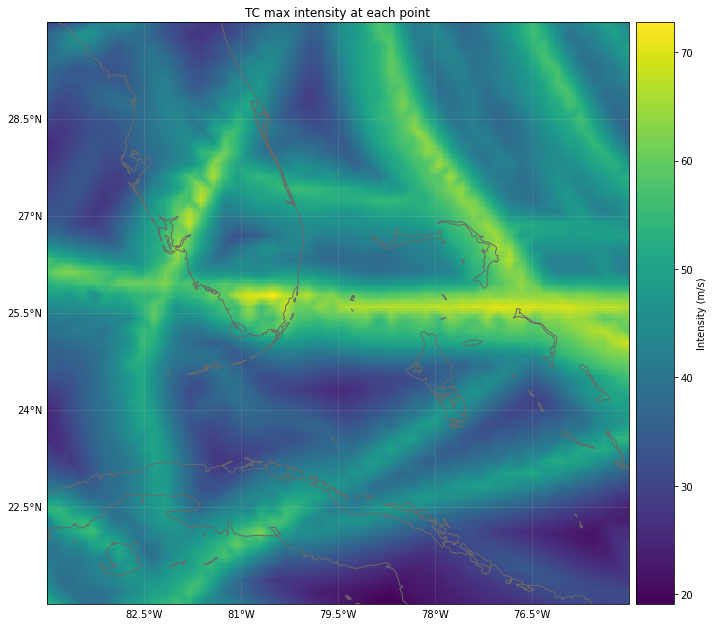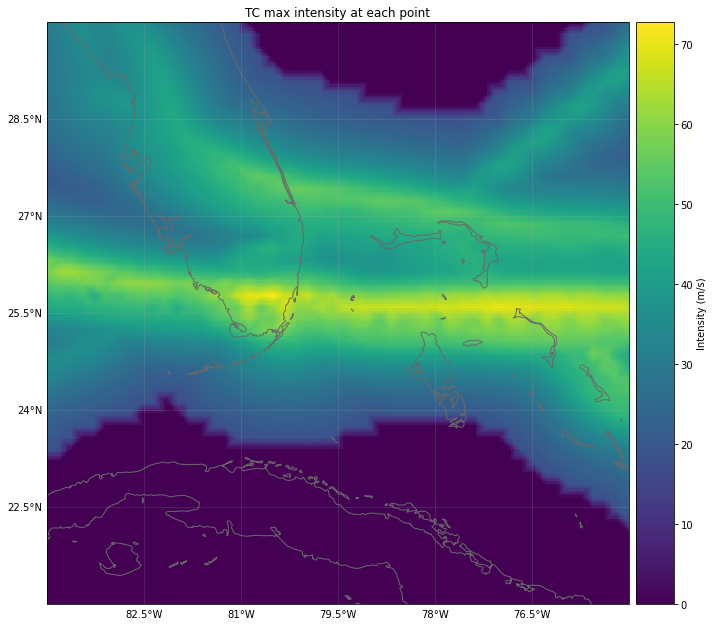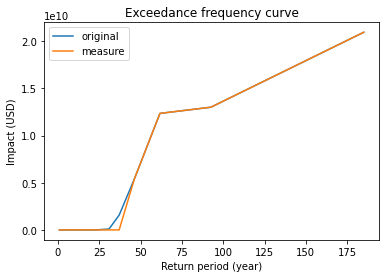# effect of exp_region_id import numpy as np from climada.entity import ImpactFuncSet, ImpfTropCyclone, Exposures from climada.entity.measures import Measure from climada.hazard import Hazard from climada.engine import ImpactCalc from climada.util import HAZ_DEMO_H5, EXP_DEMO_H5 # define measure meas = Measure( name='Building code', haz_type='TC', color_rgb=np.array([1, 1, 1]), cost=500000000, hazard_freq_cutoff=0.00455, exp_region_id=, # apply measure to points close to exposures with region_id=1 ) # impact functions impf_tc = ImpfTropCyclone.from_emanuel_usa() impf_all = ImpactFuncSet([impf_tc]) # Hazard haz = Hazard.from_hdf5(HAZ_DEMO_H5) haz.check() # Exposures exp = Exposures.from_hdf5(EXP_DEMO_H5) #exp['region_id'] = np.ones(exp.shape) exp.check() # all exposures have region_id=1 exp.plot_hexbin(buffer=1.0) # new hazard new_exp, new_impfs, new_haz = meas.apply(exp, impf_all, haz) # the cutoff has been apllied only in the region of the exposures haz.plot_intensity(0) new_haz.plot_intensity(0) # the exceddance frequency has only been computed for the selected exposures before doing the cutoff. # since we have removed the hazard of the places with exposure, the new exceedance frequency curve is zero. imp = ImpactCalc(exp, impf_all, haz).impact() imp.calc_freq_curve().plot() new_imp = ImpactCalc(new_exp, new_impfs, new_haz).impact() new_imp.calc_freq_curve().plot();  $CONDA_PREFIX/lib/python3.8/site-packages/pyproj/crs/crs.py:68: FutureWarning: '+init=<authority>:<code>' syntax is deprecated. '<authority>:<code>' is the preferred initialization method. When making the change, be mindful of axis order changes: https://pyproj4.github.io/pyproj/stable/gotchas.html#axis-order-changes-in-proj-6
return _prepare_from_string(" ".join(pjargs))

<AxesSubplot:title={'center':'Exceedance frequency curve'}, xlabel='Return period (year)', ylabel='Impact (USD)'>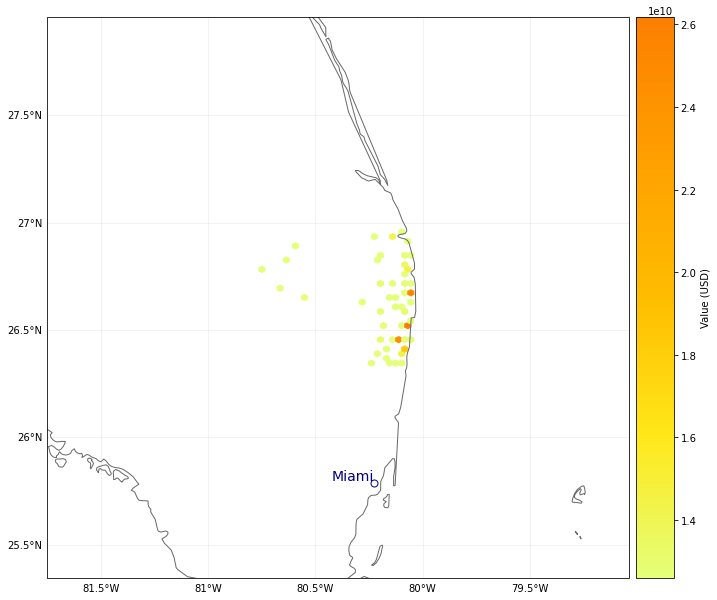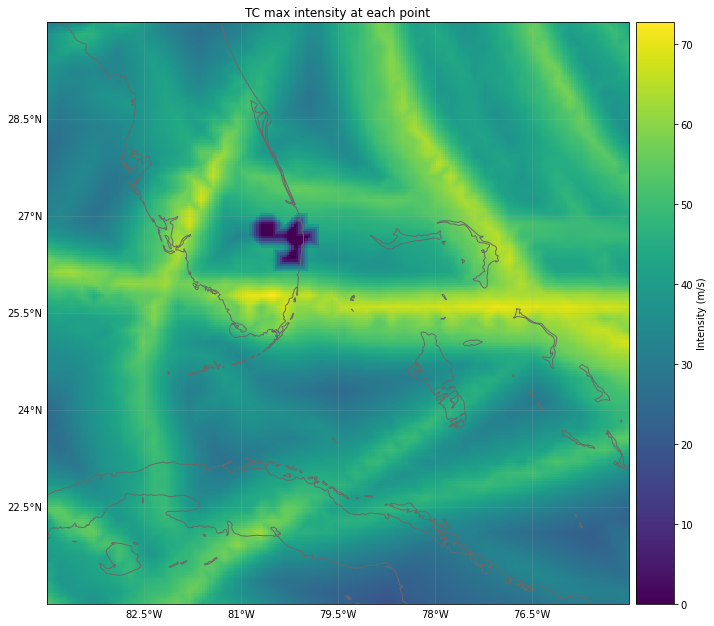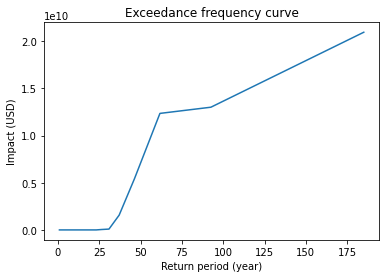# effect of risk_transf_attach and risk_transf_cover
import numpy as np
from climada.entity import ImpactFuncSet, ImpfTropCyclone, Exposures

# define measure
meas = Measure(
name='Insurance',
haz_type='TC',
color_rgb=np.array([1, 1, 1]),
cost=500000000,
risk_transf_attach=5.0e8,
risk_transf_cover=1.0e9,
)

# impact functions
impf_tc = ImpfTropCyclone.from_emanuel_usa()
impf_all = ImpactFuncSet([impf_tc])

# Hazard
haz = Hazard.from_hdf5(HAZ_DEMO_H5)
haz.check()

# Exposures
exp = Exposures.from_hdf5(EXP_DEMO_H5)
exp.check()

# impact before
imp = ImpactCalc(exp, impf_all, haz).impact()
ax = imp.calc_freq_curve().plot(label='original');

# impact after. risk_transf will be added to the cost of the measure
imp_new, risk_transf = meas.calc_impact(exp, impf_all, haz)
imp_new.calc_freq_curve().plot(axis=ax, label='measure');
print('risk_transfer {:.3}'.format(risk_transf.aai_agg))

2022-03-30 20:10:29,899 - climada.hazard.base - INFO - Reading /home/yuyue/climada/demo/data/tc_fl_1990_2004.h5
2022-03-30 20:10:30,030 - climada.entity.exposures.base - INFO - centr_ not set.
2022-03-30 20:10:30,034 - climada.entity.exposures.base - INFO - Matching 50 exposures with 2500 centroids.
2022-03-30 20:10:30,035 - climada.util.coordinates - INFO - No exact centroid match found. Reprojecting coordinates to nearest neighbor closer than the threshold = 100
2022-03-30 20:10:30,047 - climada.engine.impact - INFO - Calculating damage for 50 assets (>0) and 216 events.
2022-03-30 20:10:30,084 - climada.engine.impact - INFO - Exposures matching centroids found in centr_TC
2022-03-30 20:10:30,087 - climada.engine.impact - INFO - Calculating damage for 50 assets (>0) and 216 events.
risk_transfer 2.7e+07## MeasureSet class#

Similarly to the ImpactFuncSet, MeasureSet is a container which handles Measure instances through the methods append(), extend(), remove_measure()and get_measure(). Use the check() method to make sure all the measures have been properly set.

For a complete class documentation, refer to the Python modules docs: climada.entity.measures.measure_set.MeasureSet

# build measures
import numpy as np
import matplotlib.pyplot as plt

meas_1 = Measure(
haz_type='TC',
name='Mangrove',
color_rgb=np.array([1, 1, 1]),
cost=500000000,
mdd_impact=(1, 2),
paa_impact=(1, 2),
hazard_inten_imp=(1, 2),
risk_transf_cover=500,
)

meas_2 = Measure(
haz_type='TC',
name='Sandbags',
color_rgb=np.array([1, 1, 1]),
cost=22000000,
mdd_impact=(1, 2),
paa_impact=(1, 3),
hazard_inten_imp=(1, 2),
exp_region_id=2,
)

# gather all measures
meas_set = MeasureSet()
meas_set.append(meas_1)
meas_set.append(meas_2)
meas_set.check()

# select one measure
meas_sel = meas_set.get_measure(name='Sandbags')
print(meas_sel.name, meas_sel.cost)

Sandbags 22000000


## Read measures of an Excel file#

Measures defined in an excel file following the template provided in sheet measures of climada_python/data/system/entity_template.xlsx can be ingested directly using the method from_excel().

from climada.entity.measures import MeasureSet

# Fill DataFrame from Excel file
file_name = ENT_TEMPLATE_XLS # provide absolute path of the excel file
meas_set = MeasureSet.from_excel(file_name)
meas_set

<climada.entity.measures.measure_set.MeasureSet at 0x1f30d913b80>


## Write measures#

Measures can be writen in Excel format using write_excel() method.

from climada.entity.measures import MeasureSet

# Fill DataFrame from Excel file
file_name = ENT_TEMPLATE_XLS # provide absolute path of the excel file
meas_set = MeasureSet.from_excel(file_name)

# write file
meas_set.write_excel('results/tutorial_meas_set.xlsx')


Pickle can always be used as well:

from climada.util.save import save
# this generates a results folder in the current path and stores the output there
save('tutorial_meas_set.p', meas_set)